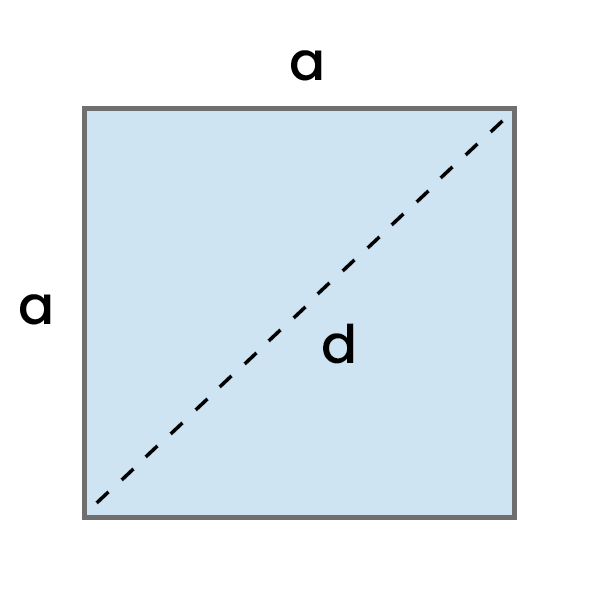# Diagonal of a Square Calculator

Enter the length of side A of the square in your chosen unit and calculate its diagonal. See the detailed step-by-step calculation process.Result:

## How to calculate Diagonal of a Square?

To calculate the diagonal of a square, multiply the length of one of its side (a) by the square root of 2 (√2).
Diagonal of a Square = a * √2 = a√2
• a - Side Length
Example: Calculate the diagonal of a square with a side length of 8 cm.

Diagonal = a * √2

Diagonal = 8 cm * √2

Diagonal = 11.3137 cm